## Filters

Sort by :
Clear All
Q

The bob of a simple pendulum has mass 2 g and charge of . It is at rest in a uniform horizontal electric field of intensity . At equilibrium, the angle that the pendulum makes with the vertical is :

(take )

• Option 1)

• Option 2)

• Option 3)

• Option 4)

Option 1)   Option 2) Option 3)   Option 4)

Determine the charge on the capacitor in the following circuit :• Option 1)

• Option 2)

• Option 3)

• Option 4)

As we know charge on capicator =  First we calculate the current in each branch                               Option 1)      Option 2)         Option 3) Option 4)

Seven capacitors , each of capacitance 2 , are to be connected in a configuration to obtain an effective capacitance of . Which of the combinations, shown in figures below, will achieve the desired value ?

• Option 1)• Option 2)• Option 3)• Option 4)Series Grouping - - wherein       Parallel Grouping - - wherein     for all capacitor. For (3 capacitor in parallel) with series with 4  capacitor Option 1)Option 2)Option 3)Option 4)

The given graph shows variation (with distance r from centre) of:• Option 1)

potential of a uniformly charged spherical shell

• Option 2)

Electric field of a uniformly spherical shell

• Option 3)

Electric field of a uniformly charged sphere

• Option 4)

Potential of a uniformly charged sphere

Inside the sphere (P lies inside the sphere) - - wherein The potential of uniformly charged spherical shell.    Option 1)potential of a uniformly charged spherical shellOption 2)Electric field of a uniformly spherical shellOption 3)Electric field of a uniformly charged sphereOption 4)Potential of a uniformly charged sphere

An electric field of 1000 V/m is applied to an electric dipole at angle of 45o . The value of electric dipole moment is 10-29 C.m. What is the potential energy of the electric dipole ?

• Option 1)

- 20 x 10-18 J

• Option 2)

- 10 x 10-29 J

• Option 3)

- 7 x 10-27 J

• Option 4)

- 9 x 10-20 J

Work done in rotation - -  Option 1)- 20 x 10-18 JOption 2)- 10 x 10-29 JOption 3)- 7 x 10-27 JOption 4)- 9 x 10-20 J

In the figure shown below the charge on the left plate of the 10 capacitor is - 30. The charge on the right plate of the 6 capacitor is:• Option 1)

• Option 2)

• Option 3)

• Option 4)

Common Potential - - Charge of 6 MFcapacitor  On right plate charge is      Option 1)Option 2)Option 3)Option 4)

Three charges Q, +q and +q are placed at vertices of a right - angle isosceles triangle as shown blow. The net electrostatic energy of the configuration is zero, if the value of Q is:• Option 1)

• Option 2)

• Option 3)

+q

• Option 4)

-2q

Potential Energy Of a System Of n Charge - - wherein For system of 3 charges. Energy of photon  Since  and as wave length increases M decreases, so Dm decreases   electron will be exite to n=4 since   Radius of atom will be 16 ao.    Option 1)Option 2)Option 3)+qOption 4)-2q

A parallel plate capacitor with plates of area 1m2 each, are at a separation of 0.1 m. If the electric field between the plates is 100 N/C , the magnitude of charge on each plate is :

(Take  )

• Option 1)

9.85 X 10-10 C

• Option 2)

8.85 X 10-10 C

• Option 3)

7.85 X 10-10 C

• Option 4)

6.85 X 10-10 C

Infinite Plane Parallel sheets of charge - If     and  EQ = 0 -   A = 1m2 d= 0.1m E = 100 N/C      Option 1)9.85 X 10-10 COption 2)8.85 X 10-10 COption 3)7.85 X 10-10 COption 4)6.85 X 10-10 C

A paraller plate capacitor having capacity 12 pF is charged by a battery to a potential difference of 10 V between its plates. The charging battery is now disconnected and a poecelain slab of dielectric constant 6.5 is slipped between the plates. The work done by the capacitor on the slab is :

• Option 1)

• Option 2)

• Option 3)

• Option 4)

Energy Stored - -        If K filled between the plates -     - wherein    (initial energy)   Since battery is disconnected charge remains same.    (final energy)           Option 1)Option 2)Option 3)Option 4)

In the circuit shown, find C if the effective capacitance of the whole circuit is to be 0.5  . All values in the circuit are in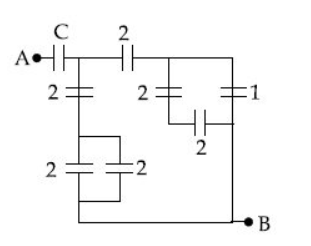• Option 1)

• Option 2)

• Option 3)

• Option 4)

Parallel Grouping - - wherein 7+3C =14C 11C =7  Option 1) Option 2)Option 3)Option 4)

Charge -q and +Q located at A and B, respectively, constitute an electric dipole.Distance AB=2a, O is the mid point of the dipole and OP is perpendicular to AB. A charge Q is places at P where OP=y and y>>2a. The charge Q experience an electrostatic force F. If Q is now moved along the equational line to P' such that  the force on Q woll be close to :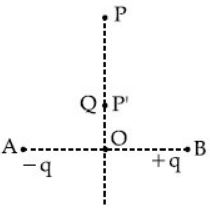• Option 1)

9F

• Option 2)

27 F

• Option 3)

F/3

• Option 4)

3F

At equatorial Point - If r >> l   - wherein Angle between Eaxial and P is 180°.   Electric field of equitorial plane of dipole At P, At P' , Option 1)9FOption 2)27 FOption 3)F/3Option 4)3F

Four equal point charges Q each are placed in the xy plane at (0,2), (4,2), (4, -2) and (0, -2). The work required to put a fifth charge Q at the ogigin of the coordinate system will be :

• Option 1)

• Option 2)

• Option 3)

• Option 4)

Potential Energy Of a System Of n Charge - - wherein For system of 3 charges.   Vorigin =            Work required to put a 5th charge Q at origin = Q Vorigin Option 1)  Option 2)  Option 3)  Option 4)

A charge Q is distributed over three concentric spherical shells of radii a,b,c (a<b<c) such that their surface charge densities are equal to one another. The total potential at a point at distance r from their common centre, where  would be:

• Option 1)• Option 2)• Option 3)• Option 4)Potential Due to 3 Concentric Spheres - The figure shows three conducting concentric shell of radii a, b and c (a < b < c) having charges Qa, Qb and Qc respectively - wherein   Potential at A; Potential at B; Potential at C;       Option 1) Option 2) Option 3) Option 4)

A Parallel plate capacitor is of area  and separation 3mm. The gap is filled with three dielectric materials of equal thickness (see figure) with dielectric constants  and . The dielectric constant of a material which when fully inserted in above capacitor, gives same capacitance would

be: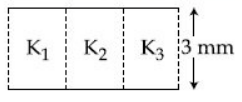• Option 1)• Option 2)• Option 3)• Option 4)If K filled between the plates -     - wherein    Option 1)Option 2)Option 3)Option 4)Two electric dipoles, A, B with respective dipole moments  are placed on the x-axis with a separation R, as shown in the figure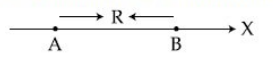The distance from A at which both of them production the same potentials is:

• Option 1)

• Option 2)

• Option 3)

• Option 4)

Electric Field and Potential due to an Electric Dipole - At axial point - wherein   let at P        Option 1)                 Option 2)Option 3)Option 4)

There is a uniform spherically symmetric surface charge density at a distance Ro from the origin. The charge distribution is initially at rest and starts expanding because of mutual repulsion. The figure that represents best the speed V(R(t)) of the distribution as a function of its instantaneous radius R(t) is :

• Option 1)• Option 2)• Option 3)• Option 4)Potential Energy Of System Of two Charge -          - wherein At any instant Total energy of charge distribution is constant       and slope of V-R curve is decreasing      Option 1)Option 2)Option 3)Option 4)

In the figure shown, after the switch 'S' is turned from position 'A' to posittion 'B' , the energy dissipated in the circuit in terms of capacitance 'c' and total charge 'Q' is :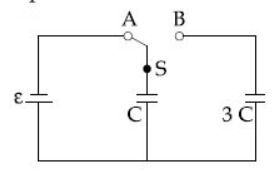• Option 1)

• Option 2)

• Option 3)

• Option 4)

Energy Stored - -                           Option 1)Option 2)Option 3)Option 4)

A parallel plate capacitor with square plates is filled with four dielectrics constant K1 , K2, K3 , K4 arranged as shown in the figure. The effective dielectric constant K will be:• Option 1)

• Option 2)

• Option 3)

• Option 4)

If K filled between the plates -     - wherein      Option 1)Option 2)Option 3)Option 4)

Charge is distributed within a sphere of radius R with a volume charge density , where A and a are constants. If Q is the total charge of this charge distribution, the radius R is:

• Option 1)

• Option 2)

• Option 3)

• Option 4)

Volume Charge distribution -  charge per unit volume. - wherein (charge on a dielect ric sphere etc)          Option 1)Option 2)Option 3)Option 4)

Determiine the electric dipole moment of the sysytem of three charges, placed on the vertices of an equilateral triangle, as shown in the figure :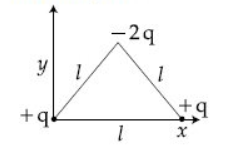• Option 1)

• Option 2)

• Option 3)

• Option 4)

Dipole moment - S.I unit - C-m or Debye     - wherein Consider this a  2 dipole as shown in figure               Option 1) Option 2)Option 3)Option 4)
Exams
Articles
Questions